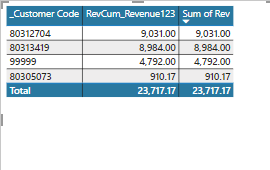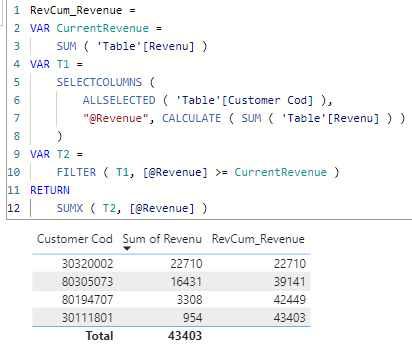cancel
Showing results for
Did you mean:Frequent Visitor

## Revverse cumulative error

RevCum_Revenue123 = VAR RunningTotal= CALCULATE([sss], FILTER(ALL(grp[Rev]),grp[Rev]>=MIN(grp[Rev])))
RETURN IF(ISBLANK([sss]),BLANK(),RunningTotal)

here [sss] = SUM(grp[Rev])when i remove customer code, it will only show the entire sum.I want to show the reverse cumulative ,
i.e in descending  order: 9031 then 9031+8984 then 9031+8984+4792.
1 ACCEPTED SOLUTIONSuper User

Please refer to attached sample file with the proposed solution``````RevCum_Revenue =
VAR CurrentRevenue =
SUM ( 'Table'[Revenu] )
VAR T1 =
SELECTCOLUMNS (
ALLSELECTED ( 'Table'[Customer Cod] ),
"@Revenue", CALCULATE ( SUM ( 'Table'[Revenu] ) )
)
VAR T2 =
FILTER ( T1, [@Revenue] >= CurrentRevenue )
RETURN
SUMX ( T2, [@Revenue] )``````
4 REPLIES 4Super User

What is the result that you're looking for?Frequent VisitorFrequent VisitorSuper User

Please refer to attached sample file with the proposed solution``````RevCum_Revenue =
VAR CurrentRevenue =
SUM ( 'Table'[Revenu] )
VAR T1 =
SELECTCOLUMNS (
ALLSELECTED ( 'Table'[Customer Cod] ),
"@Revenue", CALCULATE ( SUM ( 'Table'[Revenu] ) )
)
VAR T2 =
FILTER ( T1, [@Revenue] >= CurrentRevenue )
RETURN
SUMX ( T2, [@Revenue] )``````